• matlab设计iir低通滤波器
2022-07-14 14:04:28

# 一、登陆Matlab Online

Matlab Online网址：https://matlab.mathworks.com/
第一次需要使用邮箱注册，免费使用30天。Matlab软件本身比较大，下载安装都很麻烦，所以使用Matlab OnSTM32
更多相关内容
• Matlab设计和实现IIR低通滤波器对于智能车的车友来说，滤波器在处理比赛任务相当有效，像这样处理传感器数据q = 0.95;y = y*q + x*(1-q);如果需要更高性能的滤波器怎么办？matlab提供了IIR 滤波器设计设计工具，IIR ...

Matlab设计和实现IIR低通滤波器

对于智能车的车友来说，滤波器在处理比赛任务相当有效，像这样处理传感器数据

q = 0.95;

y = y*q + x*(1-q);

如果需要更高性能的滤波器怎么办？matlab提供了IIR 滤波器设计设计工具，IIR 滤波器的主要优点是，满足同样的性能，阶数远远低于 FIR 滤波器。

参考链接

如果对数字信号处理什么的不太会的话看的会一头雾水，下面的脚本可供参考。修改周期和设计指标为你需要的参数，运行脚本即可查看设计的滤波器的频率响应和设计滤波器的参数N，Bz，Az

% 采样周期和采样频率

T=0.001;

fs = 1/T;

%低通滤波器设计指标

Fp = 0.1*fs;%通带边界频率(hz)

Rp=1;%通带最大衰减(db)

Fs = 0.15*fs;%阻带边界频率(hz)

Rs=20;%阻带最小衰减(db)

% 0 巴特沃斯

% 1 切比雪夫1型

% 2 切比雪夫2型

% 3 椭圆滤波器

filter_type = 0;

Wp=(2/T)*tan(pi*(Fp/fs));

Ws=(2/T)*tan(pi*(Fs/fs));

if filter_type==0

[N,Wc]=buttord(Wp,Ws,Rp,Rs,'s'); % 计算滤波器阶数N和Wc

elseif filter_type==1

[N,Wc]=cheb1ord(Wp,Ws,Rp,Rs,'s'); % 计算滤波器阶数N和Wc

elseif filter_type==2

[N,Wc]=cheb2ord(Wp,Ws,Rp,Rs,'s'); % 计算滤波器阶数N和Wc

elseif filter_type==3

[N,Wc]=ellipord(Wp,Ws,Rp,Rs,'s'); % 计算滤波器阶数N和Wc

end

% Wc = Ws / ( (10^(.1*abs(Rs)) - 1)^(1/(2*N)) ) ;% 按阻带要求设计

Wc = Wp / ( (10^(.1*abs(Rp)) - 1)^(1/(2*N)) ) ;% 按通带要求设计

if filter_type==0

[z,p,k]=buttap(N); % 计算模拟滤波器原型

elseif filter_type==1

[z,p,k]=cheb1ap(N,Rp); % 计算模拟滤波器原型

elseif filter_type==2

[z,p,k]=cheb2ap(N,Rs); % 计算模拟滤波器原型

elseif filter_type==3

[z,p,k]=ellipap(N,Rp,Rs); % 计算模拟滤波器原型

end

[Bp,Ap]=zp2tf(z,p,k); % 得到归一化函数Ha(p)的系数

[Bs,As]=lp2lp(Bp,Ap,Wc); % 解归一化得模拟滤波器传递函数Ha(s)

[Bz,Az]=bilinear(Bs,As,1/T); % Ha(s)－>H(z)求数字滤波器系统函数H(z)

N

Bz

Az

[h,w] = freqz(Bz,Az,100);

subplot(2,1,1);

plot(w/(2*pi),abs(h)) ;

xlabel('f/fs');

subplot(2,1,2);

plot(w/(2*pi),20*log10(abs(h)));

xlabel('f/fs');

ylabel('幅度/db')

ylim([-100 10]);

grid on;

滤波器实现，这里的Bz和Az就是上面设计脚本生成的Bz和Az

function [yn] = user_filter(Bz,Az,xn)

%xn是输入的向量

%yn是输出的向量

nz= size(Az,2);

n= size(xn,2);

yn = zeros(1,n);

Xbuf = zeros(1,nz);

Ybuf = zeros(1,nz);

for i = 1:n

%更新Xbuf和Ybuf

for j = 1:nz-1

Xbuf(nz+1-j) = Xbuf(nz-j);

Ybuf(nz+1-j) = Ybuf(nz-j);

end

%更新差分方程

Xbuf(1) = xn(i);

yn(i) = 0;

for j = 1:nz

yn(i) = yn(i) + Bz(j)*Xbuf(j);

end

for j = 2:nz

yn(i) = yn(i) - Az(j)*Ybuf(j);

end

Ybuf(1) = yn(i);

end

end

测试，参数和上面的设计脚本一致

% 采样周期和采样频率

T=0.001;

fs = 1/T;

L=256;

Bz =[0.0002 , 0.0011 , 0.0032 , 0.0054 , 0.0054 , 0.0032 , 0.0011 , 0.0002];

Az =[1.0000 , -3.9190 , 7.0109, -7.2789 , 4.6942 , -1.8690 , 0.4236 , -0.0420];

t = 0:T:T*(L-1);

f1 = 50;%hz

f2 = 100;

f3 = 200;

f4 = 230;

x1 = sin(2*pi*f1*t) ;

x2 = 0.3*sin(2*pi*f2*t);

x3 = sin(2*pi*f3*t);

x4 = 0.4*sin(2*pi*f4*t);

xn = x1+x2+x3+x4;

%yn=filter(Bz,Az,xn);%filter是matlab的滤波器实现

yn=user_filter(Bz,Az,xn);

subplot(3,1,1)

plot(t,xn);

title('xn')

subplot(3,1,2)

plot(t,x1+x2);

title('x1+x2')

subplot(3,1,3)

plot(t,yn,'b',t+6*T,x1+x2,'r');

title('yn');

输出，大概滞后了6个周期image-20191209225045843.png

展开全文• 精品文档 MATLAB IIR数字低通滤波器设计方法 1IIR 数字低通滤波器 滤波器分两大类经典滤波器和现代滤波器 经典滤波的概念是根据傅里叶分析和变换提出的一个工程概念根据高等数学理论 任何一个满足一定条件的信号 ...
• 精品文档 引言 当今数字信号处理技术正飞速发展它不但自成一门学科更是以不同的形式渗透到其他学 科它与国民经济息息相关与国防建设紧密相连它影响和改变着我们的生产生活方式因此 受到人们普遍的关注数字化智能化和...
• 基于MATLABIIR和FIR滤波器设计-实验4 FIR数字滤波器设计.doc IIR：在MATLAB中，可以用下列函数辅助设计IIR数字滤波器：1)利用buttord和cheb1ord可以确定低通原型巴特沃斯和切比雪夫滤波器的阶数和截止频率；2)...
• IIR 滤波器设计 - 巴特沃斯滤波器 - 高通和低通滤波器
• NUMPAGES 2020级数字电子技术 课程设计 数字电子技术课程设计报告书 课题名称 基于的FIR与IIR滤波器性能比较 姓 名 学 号 院系部 电气系 专 业 电子信息工程 指导教师 刘鑫淼 孙秀婷 2012年 6月12日 基于matlab的FIR...
• 2．学会设计IIR数字滤波器； 3．根据指标要求设计数字滤波器，并进行信号的处理。 二、实验原理： 1.脉冲响应不变法 MATLAB提供impinvar(num,den,Fs)函数，可以实现利用脉冲响应不变法将模拟滤波器转换为数字滤波器...

# 一、实验目的：

1．加深对IIR数字滤波器常用指标的理解；
2．学会设计IIR数字滤波器；
3．根据指标要求设计数字滤波器，并进行信号的处理。

# 二、实验原理：

## 1.脉冲响应不变法

MATLAB提供impinvar(num,den,Fs)函数，可以实现利用脉冲响应不变法将模拟滤波器转换为数字滤波器，其调用形式为：
[numd,dend]=impinvar(num,den,Fs)
式中num和den分别表示模拟滤波器系统函数H（s）的分子多项式系统和分母多项式系数，Fs是脉冲响应不变法中的抽样频率，单位是Hz。输出变量numd和dend分别表示数字滤波器系统函数H（z）的分子多项式系统和分母多项式系数。
【例6.1】利用BW型低通滤波器及脉冲响应不变法设计满足下列指标的数字滤波器。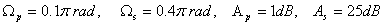程序如下：

     clear all
Wp=0.1*pi;
Ws=0.4*pi;
Ap=1;
As=25;
T=1;
Fs=1/T;
wp=Wp*Fs;
ws=Ws*Fs;
N=buttord(wp,ws,Ap,As,'s');
wc=wp/(10^(0.1*Ap)-1)^(1/2/N);
[num den]=butter(N,wc,'s');
[numd,dend]=impinvar(num,den,Fs);
w=linspace(0,pi,512);
h=freqz(numd,dend,w);
plot(w/pi,abs(h));
title('脉冲响应不变法');
%计算所设计滤波器的Ap和As
w1=[Wp,Ws];
h1=freqz(numd,dend,w1);
fprintf('Ap= %.4f\n',-20*log10(abs(h1(1))));
fprintf('As= %.4f\n',-20*log10(abs(h1(2))));


运行结果：
Ap= 0.9991
As= 30.3245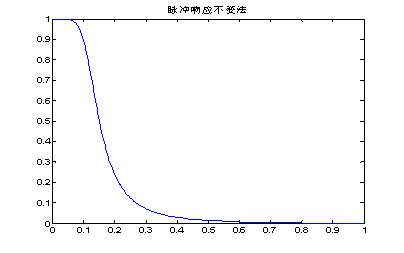图1 数字低通滤波器的幅度响应


## 2.双线性变换法

MATLAB提供bilinear(num,den,Fs)函数，可以实现利用双线性变换法将模拟滤波器转换为数字滤波器，其调用形式为：
[numd,dend]=bilinear(num,den,Fs)
式中num和den分别表示模拟滤波器系统函数H（s）的分子多项式系统和分母多项式系数，Fs=1/T。输出变量numd和dend分别表示数字滤波器系统函数H（z）的分子多项式系统和分母多项式系数。
【例6.2】利用CB1型滤波器及双线性变换法设计满足下列指标的数字高通滤波器。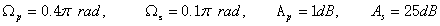程序如下：

		clear all
Wp=0.4*pi;
Ws=0.1*pi;
Ap=1;
As=25;
T=2;
Fs=1/T;
wp=(2/T)*tan(Wp/2);
ws=(2/T)*tan(Ws/2);
[N,wc]=cheb1ord(wp,ws,Ap,As,'s');
[num den]=cheby1(N,Ap,wc,'high','s');
[numd,dend]=bilinear(num,den,Fs);
w=linspace(0,pi,512);
h=freqz(numd,dend,w);
plot(w/pi,abs(h));
title('双线性变换法')
%计算所设计滤波器的Ap和As
w1=[Wp,Ws];
h1=freqz(numd,dend,w1);
fprintf('Ap= %.4f\n',-20*log10(abs(h1(1))));
fprintf('As= %.4f\n',-20*log10(abs(h1(2))));


运行结果：
Ap= 1.0000
As= 26.4153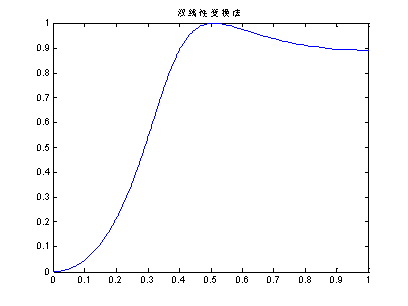图2 数字高通滤波器的幅度响应


## 3.数字滤波函数

（1）filter函数用来实现数字滤波器对数据的滤波，函数调用格式为：
y=filter(numd,dend,x)
其中，numd和dend分别为滤波器系统函数H（z）的分子和分母多项式的系数，x为滤波器的输入，y为滤波器的输出，y与x具有相同大小的向量。
（2）filtfilt函数实现零相位前后与后向结合滤波，其调用格式为：
y=filtfilt(numd,dend,x)
其中，numd和dend分别为滤波器系统函数H（z）的分子和分母多项式的系数，x为滤波器的输入，y为滤波器的输出，y与x具有相同大小的向量，这个函数实现的滤波后其输出信号与输入信号的相位一致，也就是没有改变信号波形形状。但filter函数滤波后有一些延迟，改变了信号的形状。

# 三、作业：

(1)示例代码：

clear all
Wp=0.2*pi;
Ws=0.3*pi;
Ap=1;
As=15;
T=0.01;
Fs=1/T;
wp=Wp*Fs;
ws=Ws*Fs;
N=buttord(wp,ws,Ap,As,'s');
wc=wp/(10^(0.1*Ap)-1)^(1/2/N);
[num den]=butter(N,wc,'s');
[numd,dend]=impinvar(num,den,Fs);
w=linspace(0,pi,512);
h=freqz(numd,dend,w);
plot(w/pi,abs(h));
title('脉冲响应不变法');
%计算所设计滤波器的Ap和As
w1=[Wp,Ws];
h1=freqz(numd,dend,w1);
fprintf('Ap= %.4f\n',-20*log10(abs(h1(1))));
fprintf('As= %.4f\n',-20*log10(abs(h1(2))));


（2）运行结果：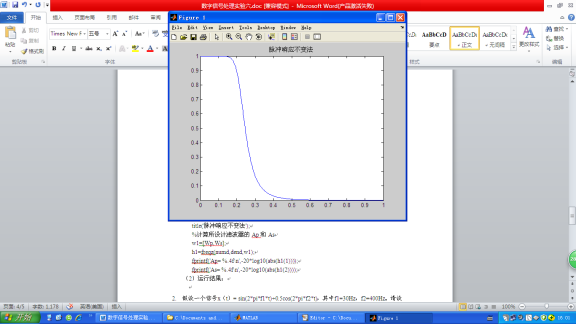2.假设一个信号x（t）= sin(2pif1t)+0.5cos(2pif2t)，其中f1=30Hz，f2=400Hz。请设计一个IIR数字滤波器，将f2滤除掉。请写出程序，并画出原信号与原信号通过滤波器的输出信号的图形。（数字低通滤波器）
（1）示例代码：

clear all
Wp=0.075*pi;
Ws=1*pi;
Ap=1;
As=25;
T=0.01;
Fs=1/T;
wp=Wp*Fs;
ws=Ws*Fs;
N=buttord(wp,ws,Ap,As,'s');
wc=wp/(10^(0.1*Ap)-1)^(1/2/N);
[num den]=butter(N,wc,'s');
[numd,dend]=impinvar(num,den,Fs);

w=linspace(0,pi,512);
h=freqz(numd,dend,w);
plot(w/pi,abs(h));
title('脉冲响应不变法');
%计算所设计滤波器的Ap和As
w1=[Wp,Ws];
h1=freqz(numd,dend,w1);
fprintf('Ap= %.4f\n',-20*log10(abs(h1(1))));
fprintf('As= %.4f\n',-20*log10(abs(h1(2))));
%信号传输
fs=800;
dt=1/fs;   %模拟信号采样间隔
f1=30;f2=400;
t=0:dt:1;
x=sin(2*pi*f1*t)+0.5*cos(2*pi*f2*t);
y=filter(numd,dend,x); % 模拟输出
L=length(x);
x1=fftshift(fft(x));
ff=(-L/2:L/2-1)*fs/L;
y1=fftshift(fft(y));
figure(2)
subplot(2,2,1)
plot(t,x);
xlabel ('(a)输入信号');
subplot(2,2,2)
plot(ff,abs(x1));
xlabel ('(b)输入信号频谱');
subplot(2,2,3)
plot(t,y);
xlabel('(c)输出信号');
subplot(2,2,4)
plot(ff,abs(y1));
xlabel ('(d)输出信号频谱'


（2）运行结果：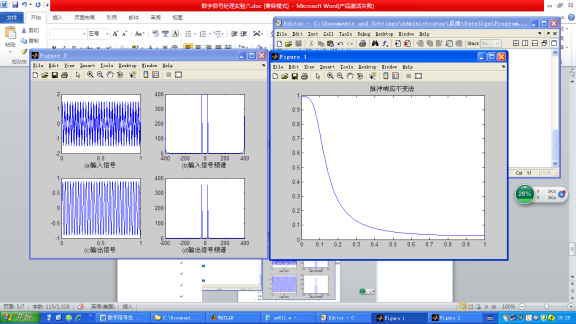3.假设一个信号x（t）= sin(2pif1t)+0.5cos(2pif2t)，其中f1=10Hz，f2=100Hz。请设计一个IIR数字滤波器能把f1滤除掉，请写出程序，并画出原信号与原信号通过滤波器的输出信号的图形。
（1）示例代码：

clear all
Wp=0.1*pi;
Ws=0.2*pi;
Ap=1;
As=25;
T=1;
Fs=1/T;
wp=(2/T)*tan(Wp/2);
ws=(2/T)*tan(Ws/2);
[N,wc]=cheb1ord(wp,ws,Ap,As,'s');
[num den]=butter(N,wc,'s');
[numd,dend]=impinvar(num,den,Fs);
w=linspace(0,pi,512);
h=freqz(numd,dend,w);
plot(w/pi,abs(h));
title('脉冲响应不变法');
%计算所设计滤波器的Ap和As
w1=[Wp,Ws];
h1=freqz(numd,dend,w1);
fprintf('Ap= %.4f\n',-20*log10(abs(h1(1))));
fprintf('As= %.4f\n',-20*log10(abs(h1(2))));
%信号传输
fs=800;
dt=1/fs;   %模拟信号采样间隔
f1=10;f2=100;
t=0:dt:1;
x=sin(2*pi*f1*t)+0.5*cos(2*pi*f2*t);
y=filter(numd,dend,x); % 模拟输出
L=length(x);
x1=fftshift(fft(x));
ff=(-L/2:L/2-1)*fs/L;
y1=fftshift(fft(y));
figure(2)
subplot(2,2,1)
plot(t,x);
xlabel ('(a)输入信号');
subplot(2,2,2)
plot(ff,abs(x1));
xlabel ('(b)输入信号频谱');
subplot(2,2,3)
plot(t,y);
xlabel('(c)输出信号');
subplot(2,2,4)
plot(ff,abs(y1));
xlabel ('(d)输出信号频谱');


（2）运行结果：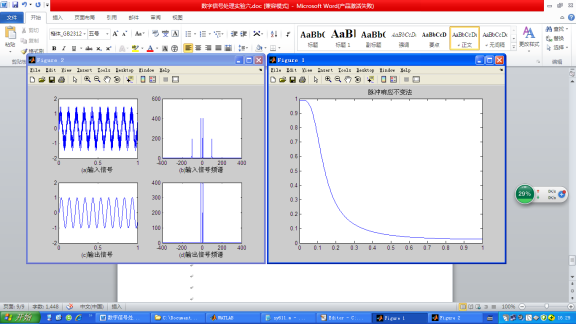4.假设一个信号x（t）=sin(2pif1t)+sin(2pif2t)+sin(2pif3*t),其中f1=200Hz，f2=1500Hz，f3=2900Hz。请设计一个IIR数字滤波器能把f2保留，请写出程序，并画出原信号与原信号通过滤波器的输出信号的图形。
（1）示例代码：

clear all
Wp=[0.2*pi 0.4*pi];
Ws=[0.1*pi 0.5*pi];
Ap=1;
As=25;
T=1;
Fs=1/T;
wp=(2/T)*tan(Wp/2);
ws=(2/T)*tan(Ws/2);
[N,wc]=cheb1ord(wp,ws,Ap,As,'s');
wc=wp/(10^(0.1*Ap)-1)^(1/2/N);
[num den]=butter(N,wc,'s');
[numd,dend]=impinvar(num,den,Fs);
w=linspace(0,pi,512);
h=freqz(numd,dend,w);
plot(w/pi,abs(h));
title('脉冲响应不变法');
%计算所设计滤波器的Ap和As
w1=[Wp,Ws];
h1=freqz(numd,dend,w1);
fprintf('Ap= %.4f\n',-20*log10(abs(h1(1))));
fprintf('As= %.4f\n',-20*log10(abs(h1(2))));
%信号传输
fs=800;
dt=1/fs;   %模拟信号采样间隔
f1=200;f2=1500;f3 = 2900;
t=0:dt:1;
x=sin(2*pi*f1*t)+sin(2*pi*f2*t)+sin(2*pi*f3*t);
y=filter(numd,dend,x); % 模拟输出
L=length(x);
x1=fftshift(fft(x));
ff=(-L/2:L/2-1)*fs/L;
y1=fftshift(fft(y));
figure(2)
subplot(2,2,1)
plot(t,x);
xlabel ('(a)输入信号');
subplot(2,2,2)
plot(ff,abs(x1));
xlabel ('(b)输入信号频谱');
subplot(2,2,3)
plot(t,y);
xlabel('(c)输出信号');
subplot(2,2,4)
plot(ff,abs(y1));
xlabel ('(d)输出信号频谱');


（2）运行结果：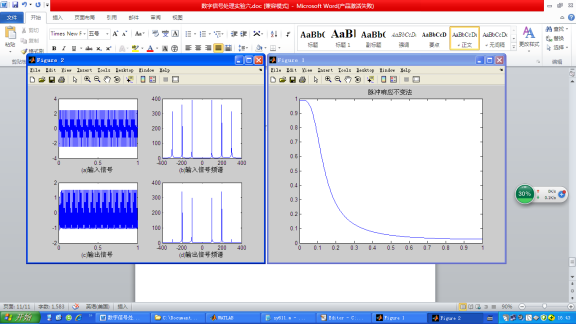# 更多相关文章点这里哦

数字信号处理----全套Matlab实验报告

通信工程(信息类，电子类，电气工程，自动化，计算机，软件工程，机电，等相关专业)全套学习指导答疑资料qq群:1007576722

展开全文算法 数字信号处理
• 精品文档 精品文档 PAGE PAGE #欢迎下载 MATLAB IIR数字低通滤波器设计方法 1 IIR数字低通滤波器 滤波器分两大类经典滤波器和现代滤波器 经典滤波的概念是根据傅里叶分析和变换提出的一个工程概念根据高等数学理论...
• IIR滤波器设计指标（specifications）5.1 滤波器的频率响应特性5.2 模拟滤波器类型5.3 IIR滤波器结构5.3.1 Direct Form IIR structure5.3.2 Direct Form I IIR structure5.3.3 Direct Form II IIR structure6....

目录

1. 概要

2. IIR 和 FIR 滤波器的定义

3. IIR滤波器和FIR滤波器的优缺点对比

5. IIR滤波器设计指标（specifications）

5.1 滤波器的频率响应特性

5.2 模拟滤波器类型

5.3 IIR滤波器结构

5.3.1 Direct Form IIR structure

5.3.2 Direct Form I IIR structure

5.3.3 Direct Form II IIR structure

6. 基于Matlab的SOS IIR滤波器设计例

# 1. 概要

本文概要性地介绍IIR滤波器设计的基础知识，以及基于Matlab进行IIR滤波器设计的示例。

# 2. IIR 和 FIR 滤波器的定义

数字滤波器，顾名思义，是一种离散时间系统（ discrete-time systems），用于对采样信号进行算法性的处理，以改变（比如说减弱或者增强等）输入信号中某种特定的属性。

比如说，从频域的角度来看，数字滤波器可以用来使得输入信号的某些频率范围的分量通过，而使得另外一些频率范围的分量被阻塞（衰减），或者使得某些频率范围的信号的相位发生所希望的改变。

从时域的角度来看，数字滤波器对于输入信号中的动态变化的响应可以用系统冲激响应来表征。在频域中，滤波器的特性则由系统传递函数（transfer function of the system）来刻画。

冲激响应（impulse response）总是一个无限长度的实现信号序列，其中，每个（采样）时刻的值可以是零也可以是非零。数字滤波器的冲激响应中的非零值通常被称为抽头（Taps）。根据冲激响应中抽头数（即非零值的个数）是有限的还是无限的，数字滤波器可以分为两类：无限冲激响应（Infinite Impulse Response ：IIR) 滤波器；有限冲激响应（Finite Impulse Response ：FIR) 滤波器。

IIR滤波器的冲激响应中包含无限个非零值，而FIR滤波器的冲激响应中只包含有限个非零值。

注：以上仅为一种看待数字滤波器分类的观点。另一种等价的观点是看冲激响应的非零区间的覆盖范围（英文文献中常用support这个词来表示）是有限长的还是无限长的。

从结构上看，IIR滤波器和FIR滤波器有一个显著的差异就是IIR滤波器有反馈部分，而FIR滤波器没有反馈部分。

## 3. IIR滤波器和FIR滤波器的优缺点对比

当需要设计一个数字滤波器时，需要在IIR滤波器和FIR滤波器两种类型之间进行折衷考虑，在能够满足系统设计需求的前提条件下在实现代价、稳定性等方面取得最好的折衷。以下列举IIR滤波器的优缺点，显而易见的是它们分别对应FIR滤波器的缺点和优点。

•         • 获取等价的特性的前提下，IIR滤波器的运算负荷更低。更通俗一点说就是IIR所需要的抽头数更少
•         • IIR滤波器的处理延迟（更正式的提法是群时延 group delay）更低（这个当然也是与抽头数少直接相关联的）

•         • 系统稳定性更难以保证。但是通过仔细的选择零点和极点的位置及搭配可以有效地缓解这个问题，滤波器设计工具可以提供关于零极点选择的有效帮助。与之相对，FIR滤波器是无条件稳定的。
•         • 系统的相位响应更难以控制。FIR滤波器通过采用具有对称性的抽头设计可以获得线性相位响应，但是IIR则比较难以控制其相位响应，更不用谈什么线性相位响应。
•         • 对数值计算的误差更加敏感。数字滤波器通常在某种形式的计算机中执行运算，其数值表示精度是有限的（即便浮点运算也是有限精度的），有限精度就必然带来运算误差，而IIR滤波器由于其存在反馈路径使得误差会累积传递，所以相比FIR滤波器更加容易受到有限精度运算的影响。
•         • IIR滤波器设计的数学流程更加复杂、模糊。与之相对的是，FIR滤波器设计存在确定性的最优的设计流程。所幸的是像Matlab、Pyton Scipy等都提供了优良的滤波器设计工具缓解了这一问题。

如前所述，数字滤波器的在频域上的响应可以由它的传递函数（transfer function）来刻画。IIR滤波器的传递函数表示为一个z变换分式，其中分子对应于IIR滤波器的前向（feed-forward）部分，包含了系统的零点（zeros）；分母对应于IIR滤波器的反馈（feedback）部分，包含了系统的极点（poles）。IIR的极点的个数表示滤波器的阶数（order），零、极点的位置则决定了系统的频率响应。注意：FIR的传递函数没有分母（或者说分母为1）,因此其阶数由零点的个数（或者更直观地说表示传递函数的多项式的阶数）决定。但是对于IIR滤波器来说，如果分子多项式的阶数高于分母多项式的阶数时，总是可以将其进行分解成两部分的级联，其中一部分是分子多项式的阶数不高于分母多项式的阶数的IIR滤波器，另部分则仅包含单纯的延时处理。因此我们可以将IIR滤波器的讨论仅限于其传递函数的分子多项式的阶数不高于分母多项式的阶数的情况。

# 5. IIR滤波器设计指标（specifications）

IIR滤波器设计指标（设计要求）通常从以下几个方面来定义：

•         1. 频率响应特性
•         2. 模拟滤波器模板
•         3. 结构 structure

## 5.1 滤波器的频率响应特性

常见的滤波器的幅频响应有以下四类（本文不讨论如全通滤波器希尔伯特滤波器等之类的特殊滤波器）：低通滤波器；高通滤波器；带通滤波器；带阻滤波器。四种幅频响应特性分别如下图所示。其中带通滤波器和带阻滤波器均可以由一个低通滤波器和一个高通滤波器的级联而得。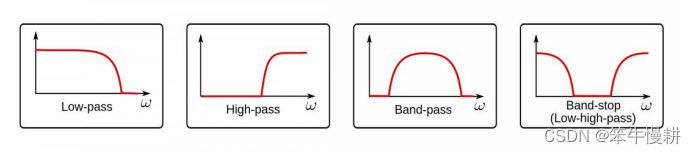图1. 四种幅频响应特性

由于IIR滤波器通常不具有线性相位，因此其应用场景也通常就是不要求相位响应的地方。因此IIR滤波器的频率响应指标定义通常都只关注幅频响应。

确定了以上幅频响应类型后，相应地需要确定通带带宽及起止频率、阻带衰减、通带波纹等指标要求。

以一个低通滤波器的设计要求为例，其设计指标要求可以表示为如下图所示：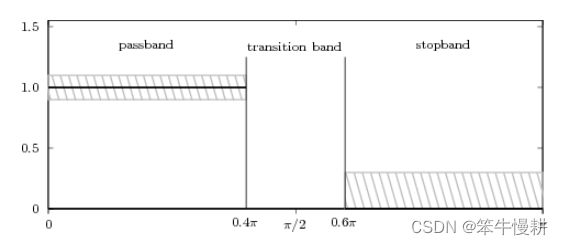图2 典型的低通滤波器设计指标要求

以下三图则分别是满足（最后一种）和不满足（前两种）以上要求的几种情况。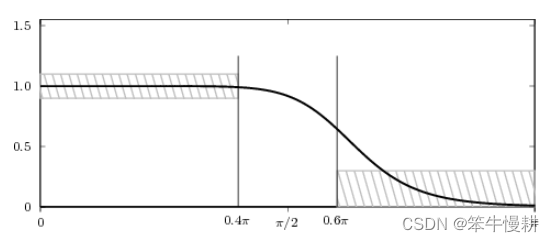图2-1 不满足设计要求的例子（阻带衰减有一部分不满足）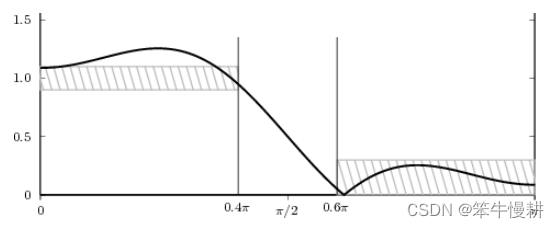图2-2 不满足设计要求的例子（通带波纹不满足）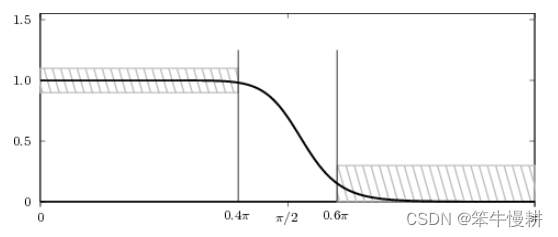图2-3 满足（甚至是超过了）设计要求的例子

## 5.2 模拟滤波器类型

IIR滤波器设计不像FIR滤波器设计那样有成熟的优化算法流程可用，所以通常采取参考模拟滤波器模板的设计流程的方式进行，通过调整模拟滤波器参数值的方式来满足所需要的幅度和相位特性。Matlab等信号处理工具提供了很好的设计IIR滤波器的工具。 通常来说，基于Matlab设计IIR滤波器的第一步就是先确定模拟滤波器模板类型。

常见的模拟滤波器有以下4种类型。

巴特沃斯（Butterworth）滤波器，其主要特性如下：

• 通带和阻带没有波纹（ripple）

• 通带到阻带的滚降（roll-off）最平缓（换言之，过渡带不陡峭）

• 相位失真适中

切比雪夫I型（Chebyshev I）滤波器，其主要特性如下：

• 通带有波纹（Ripple），阻带平坦（无波纹）

• 过渡带滚降特性比巴特沃斯滤波器要陡峭

• 群时延特性较差，即相位失真比巴特沃斯严重

切比雪夫II型（Chebyshev II, Inverse Chebyshev I）滤波器 ，其主要特性如下：

• 通带平坦（无波纹），阻带有波纹

• 过渡带滚降特性介乎于巴特沃斯滤波器与切比雪夫II型之间

椭圆型（Elliptical）滤波器，其主要特性如下：

• 通带和阻带都有波纹

• 过渡带滚降特性是（这四种之中）最陡峭的

以下图中显示了以上四种低通模拟滤波器的频域响应（假定相同的带宽和阻带衰减等设计指标，with the same specifications）。注意这些滤波器在通带、阻带波纹以及过渡带陡峭度方面的差异对比。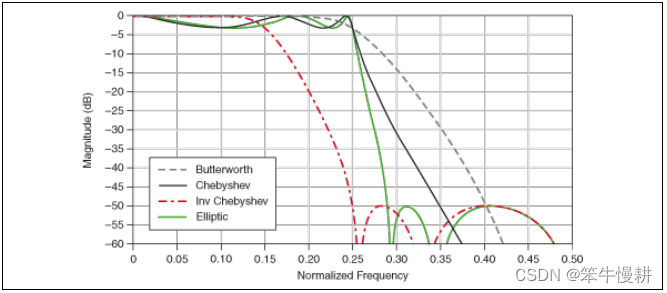图3 相同设计指标条件下四种模拟滤波器的频率响应的对比

## 5.3 IIR滤波器结构

### 5.3.1 Direct Form IIR structure

一种直观的IIR滤波器的差分方程的实现结构如下所示，这种结构被称为Direct Form结构。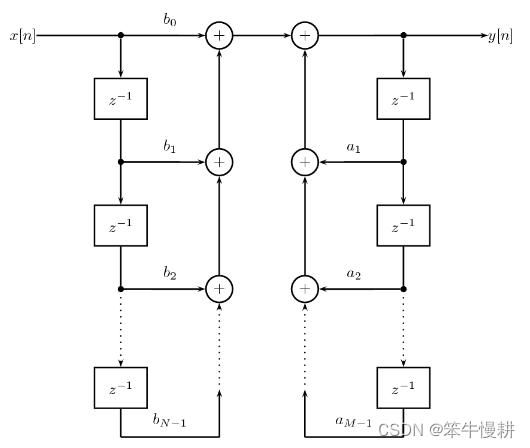图4 Direct Form structure of an IIR filter.

### 5.3.2 Direct Form I IIR structure

IIR滤波器的前馈（feed-forward）部分和反馈（feedback）部分的处理是顺序可交换的，将Direct Form的结构中前馈部分和反馈部分顺序交换一下即可得到如下结构：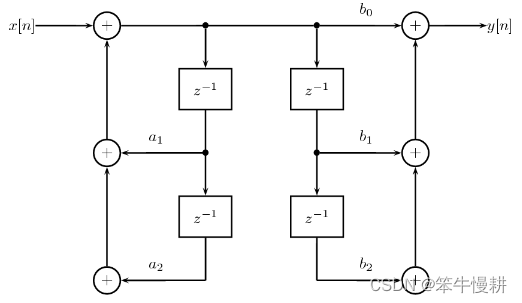图5  Direct Form I structure

乍一看，这种交换似乎并没有什么好处。但是，仔细一看可以发现现在排在中间的两排延迟链是重复的，因此是可以融合到一起的，由此我们可以得到Direct Form II结构如下所示。

### 5.3.3 Direct Form II IIR structure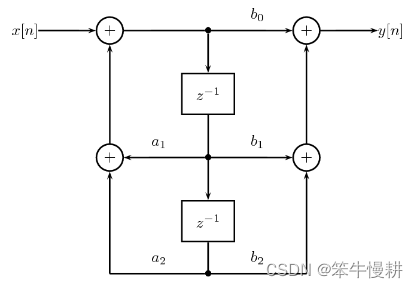图6  Direct Form II structure

这种结构的特征是延迟单元使用量最少（ the minimum number of delay elements），因而也就称为最常用的IIR滤波器实现结构。

通过将图6这种结构级联起来就可以得到更高阶的传递函数的实现，如下图所示：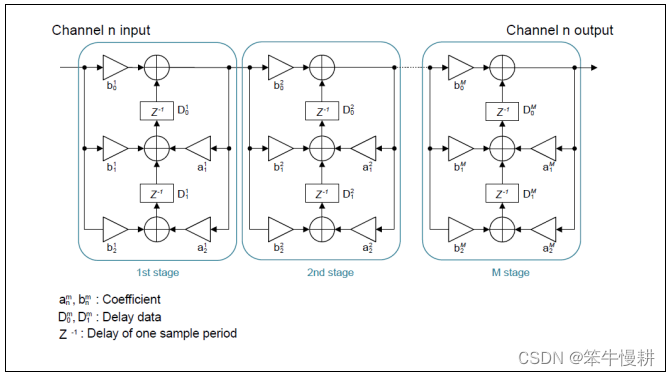# 6. 基于Matlab的SOS IIR滤波器设计例

N = 10;
Fc = 0.4;
[b,a] = butter(N,Fc);

% Create a dsp.IIRFilter object and assign the designed coefficients to the Numerator and the Denominator properties of the object.

iir = dsp.IIRFilter('Numerator',b,'Denominator',a)

% Use the sos function to convert the IIR filter object into a biquadratic filter that contains the corresponding SOS sections.

% You can access the SOS matrix and the scale value properties of the biquadratic filter.

% To verify, compare these values to the values obtained using the tf2sos function.
[sMatrix2,g] = tf2sos(b,a)
isequal(sMatrix,sMatrix2)
isequal(sValues(1),g)

除了butter()以外，还有cheby1(), cheby2(),ellip()分别用于设计前面提到的几种经典的模拟滤波器的对应的离散版本IIR滤波器的传递函数。 这些函数都有一个参数指定是设计低通特性还是高通特性。如下例所示。

生成了sos matrix以后，可以直接调用sosfilt()函数以sos matrix为参数对输入数据进行滤波。

load chirp.mat         % 加载chirp数据。这是matlab内置的例子数据。
t = (0:length(y)-1)/Fs;
[zhi,phi,khi] = butter(7,0.48,'high'); % 'high'参数指定生成高通滤波器
soshi = zp2sos(zhi,phi,khi);
freqz(soshi)
outhi = sosfilt(soshi,y); %利用所生成的sos矩阵，调用sosfilt()进行滤波

figure
subplot(2,1,1)
plot(t,y)
title('Original Signal')
ys = ylim;

subplot(2,1,2)
plot(t,outhi)
title('Highpass-Filtered Signal')
xlabel('Time (s)')
ylim(ys)

高通滤波器的效果如下，肉眼看不出什么太大的差异。这个不足为奇，因为chirp信号本来就主要是由高频成分构成。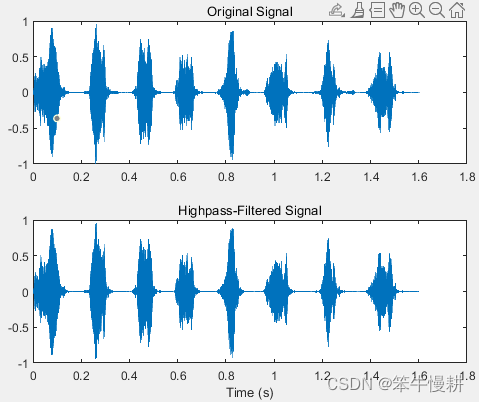以下为低通滤波示例。

[zlo,plo,klo] = butter(7,0.48); % 没有指定类型参数，缺省为低通滤波器
soslo = zp2sos(zlo,plo,klo);

outlo = sosfilt(soslo,y);

subplot(2,1,1)
plot(t,y)
title('Original Signal')
ys = ylim;

subplot(2,1,2)
plot(t,outlo)
title('Lowpass-Filtered Signal')
xlabel('Time (s)')
ylim(ys)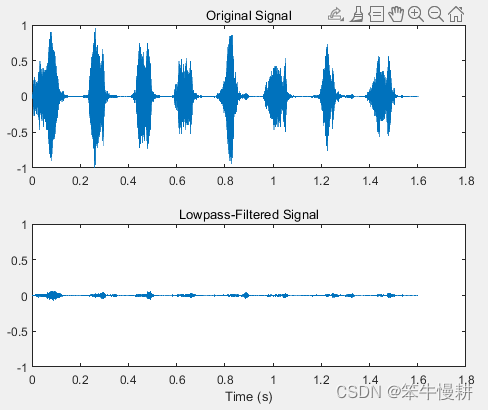可以看出，经过低通滤波后，几乎没有剩下什么了。从另一个角度印证了chirp信号主要由高频成分构成，低频成分基本上都是噪声了。

参考文献：

展开全文• IIR 数字低通滤波器(用MATLAB GUI设计.内附源码) 使用MATLAB GUI 制作可以更改参数的IIR数字低通滤波器,已生成可独立执行的EXE格式文件.里面有工程文件的原码FIG.用户可以根据自已的要求更改界面,再生成EXE格式，...
• IIR数字滤波器是数字信号处理的重要工具之一。利用Matlab信号处理工具箱和使用切比雪夫法设计IIR数字滤波器,并进行仿真。仿真结果表明,设计过程简单方便。
• 1. 设计IIR巴特沃斯低通滤波器 2. 将滤波器用于加噪声信号的处理 3. 改变参数指标比较不同 4. 实验结果与分析 （1）滤波器频率特性如图 输入信号特性如图: 经过滤波器之后信号特性如图: ...
• IIR 数字低通滤波器(用MATLAB GUI设计.内附源码) 使用MATLAB GUI 制作可以更改参数的IIR数字低通滤波器,已生成可独立执行的EXE格式文件.里面有工程文件的原码FIG.用户可以根据自已的要求更改界面,再生成EXE格式
• matlab设计IIR滤波器源程序(1)IIR一阶低通滤波器 P576clear;fi=1;fs=10;Gc2=0.9;wc=2*pi*fi/fs;omegac=tan(wc/2);alpha=(sqrt(Gc2)/sqrt(1-Gc2))*omegac;a=(1-alpha)/(1+alpha);b=(1-a)/2;w=0:pi/300:pi;Hw2=...
• 由于电力系统中非线性电子...目前有源电力滤波器(APF)是治理电网谐波污染的一种有效手段，APF的补偿原理是实时产生一个与系统中的无功和谐波电流大小相等、方向相反的补偿电流，用以抵消非线性负载产生的无功和谐波...
• 利用matlab设计巴特沃斯低通滤波器 三峡大学 课 程 设 计 报 告 专业班级 20091421 课 程 数字信号处理课程设计 学 号 2009142116 学生姓名 姜祥奔 指导教师 王露 2012年 5 月 平时成绩(20%) 报告成绩(40%) 答辩成绩...
• 基于MATLABIIR数字低通滤波器设计.pdf
• 基于 matlab低通滤波器 摘要:调用 MATLAB 信号处理工具箱中滤波通过观察滤波器输入输出信号的时域波形及其频谱建立数字滤波的概 念应用最广泛的是双线性变换法基本设计过程是先将给定的数字滤波器的指标转换成...
• 基于matlabIIR滤波器设计-基于matlabIIR数字滤波器设计-刘智.doc 全面的介绍IIR滤波器的设计
• 基于MatlabIIR数字低通滤波器设计方法.pdf
• 基于MATLABIIR数字低通滤波器设计.rar
• 基于MATLAB GUI的IIR数字滤波器语音信号去噪处理平台的设计与实现 代码而已
• 本程序通过直接法，实现了给定参数的IIR低通滤波器设计。 本程序所设计的滤波器的参数与Matlab校准过。 将需要的数据输入.dat文件，实现自动处理。其输出与Matlab一致。 详细的设计过程，参看博客。 ...
• MATLAB 实现低通高通带通，FIR，IIR滤波器
• 基于MATLABIIR和FIR滤波器设计-实验5 基于MATLAB的数字滤波器设计.doc IIR：在MATLAB中，可以用下列函数辅助设计IIR数字滤波器：1)利用buttord和cheb1ord可以确定低通原型巴特沃斯和切比雪夫滤波器的阶数和截止...
• 附件为8阶（2*4）巴特沃兹iir低通滤波器c语言实现代码 无平台限值 可直接运行 详细滤波器系数已写在文件头 其中系数数组b、a由matlab的fdatool计算生成，具体系数对应方法可参考 ...
• 设计数字低通滤波器matlab 实现 一实验目的掌握 IIR 数字低通滤波器设计方法 二实验原理 1滤波器的分类 滤波器分两大类经典滤波器和现代滤波器 经典滤波器是假定输入信号 x(n) 中的有用成分和希望取出的成分...
• 利用matlab设计FIR低通滤波器和IIR低通滤波器。编译通过。...

# matlab设计iir低通滤波器matlab 订阅